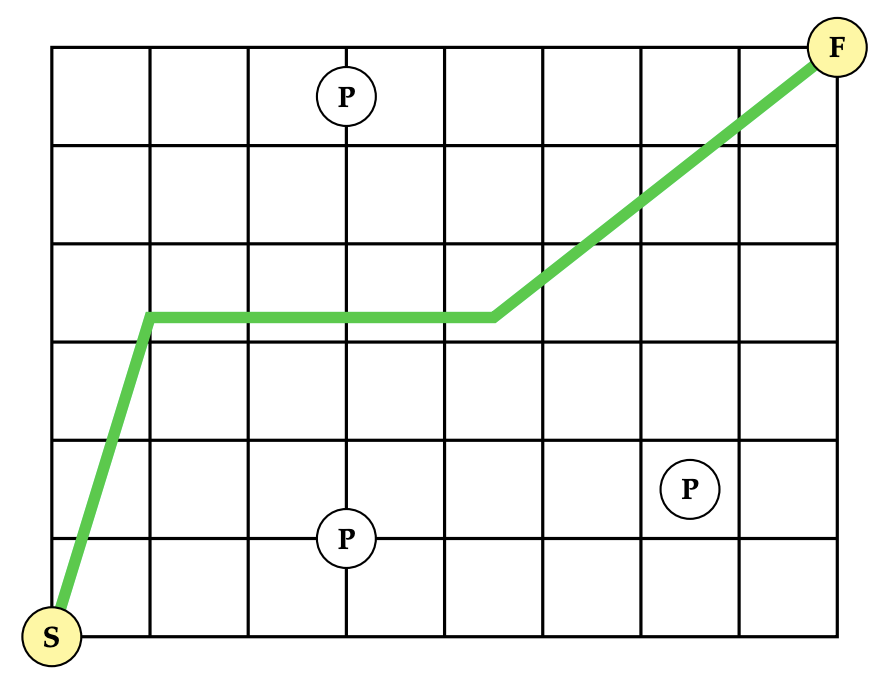시간 제한 메모리 제한 제출 정답 맞은 사람 정답 비율
1 초 512 MB 16 10 8 57.143%

## 문제

The past year has been difficult, with a virus spreading among the population. Fortunately, Alice knows that one of the keys to be healthy is to keep a safe distance from other people.

Alice is currently in a closed room, represented in the $2D$ plane, with width $X$ and height $Y$. There are $N$ other people inside the room, and we're given their $(x_i, y_i)$ coordinates.

We consider Alice and the $N$ people as points in the $2D$ plane. Alice's initial position is $(0, 0)$ and she wants to move to the exit at position $(X, Y)$. She can move freely in any direction inside the room, but can not step outside the room bounds.

Find the maximum distance Alice can keep from other people while moving from $(0, 0)$ to $(X, Y)$.

## 입력

The input begins with one line containing two space-separated integers, $X$ and $Y$, where $X$ is the width, and $Y$ is the height of the room.  The second line consists of a single integer $N$, the number of people in the room.  Then $N$ lines follow, each of them consisting of two floating-point numbers $x_i$ and $y_i$, the coordinates of the $i^{th}$ person in the room.

## 출력

The output consists of a single value $d$, the maximum safe distance,  as a floating-point number.

An additive or multiplicative error of $10^{-5}$ is tolerated: if $d$ is the answer, any number either within $[d - 10^{-5}; d + 10^{-5}]$ or within $[(1 - 10^{-5})d ;(1 + 10^{-5})d]$ is accepted.

## 제한

• $1 \le X, Y \le 1\,000\,000$
• $1 \le N \le 1\,000$
• $0 \le x_i \le X$
• $0 \le y_i \le Y$

## 예제 입력 1

8 6
3
3 1
3 5.5
6.5 1.5


## 예제 출력 1

2.250000


Alice can keep a distance of 2.25 from every other person, and this is the best she can do. The picture below shows a possible path (in green).## 출처• 문제를 만든 사람: Miguel Oliveira